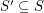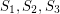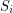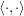Importance: Medium ✭✭
 Author(s): Noel, Jonathan A.
 Subject: Geometry
 Keywords: Partitioning projective plane
 Posted by: Jon Noel on: August 27th, 2013

Throughout this post, by projective plane we mean the set of all lines through the origin in.

Definition   Say that a subsetof the projective plane is octahedral if all lines inpass through the closure of two opposite faces of a regular octahedron centered at the origin.
Definition   Say that a subsetof the projective plane is weakly octahedral if every setsuch thatis octahedral.
Conjecture   Suppose that the projective plane can be partitioned into four sets, sayandsuch that each setis weakly octahedral. Then eachis octahedral.

Also, see the posting on mathoverflow.

There is an equivalent definition of the "weakly octahedral" condition which may be useful.

Lemma   A subsetof the projective plane is weakly octahedral if for any three lines inand any three vectorsandwhich span these lines, we havewhereis the standard (dot) inner product on.

The fact thatandpartition the projective plane seems to be important. Here is an example of a weakly octahedral set that is not octahedral: Fix any vectorand letbe the set of all lines which are spanned by vectors which meetat an angle strictly less than.

This question came up while working on another problem posted to this site: Circular colouring the orthogonality graph. It is possible that a solution to the problem stated here can be applied to solve this problem. Moreover, it may be useful in proving that the real orthogonality graph (defined in the other posting) has (essentially) only one proper-colouring.

## Bibliography

* indicates original appearance(s) of problem.